## Monday, October 31, 2016

### Costume (Math) Department

(Click on the comic if you can't see the full image.)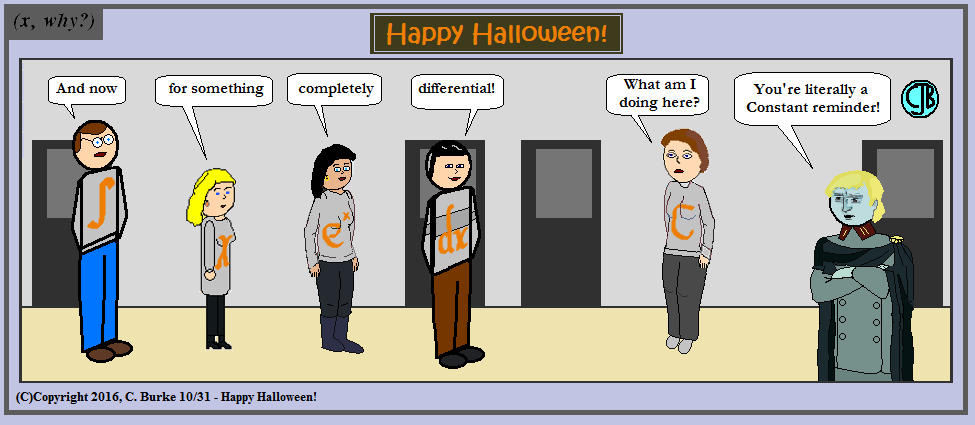We all need Constant reminders to Be Safe, and Have Fun. And add a Constant.

Happy Halloween.

So what's you're opinion of the Math Horror Movies?

Come back often for more funny math and geeky comics.## Friday, October 28, 2016

### Math Horror Movies: Lost Solutions

(Click on the comic if you can't see the full image.)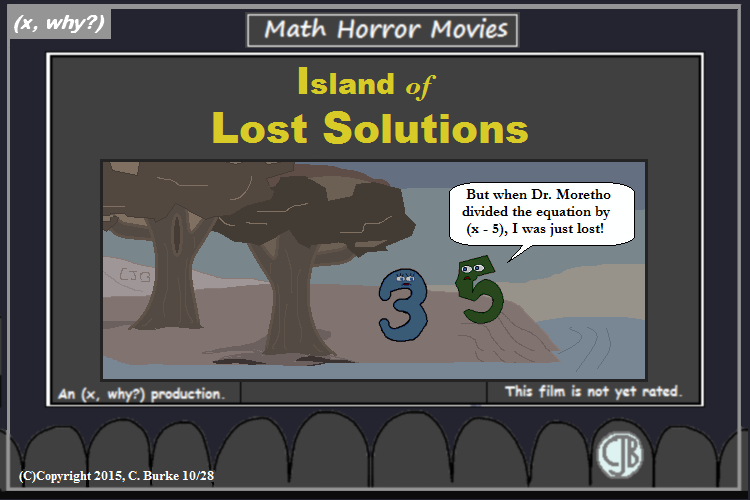Today's Math Lesson is brought to you by the Dr. Moretho's House of Pain.

What is the Law?

Come back often for more funny math and geeky comics.## Wednesday, October 26, 2016

### Math Horror Movies: 1000 i's

(Click on the comic if you can't see the full image.)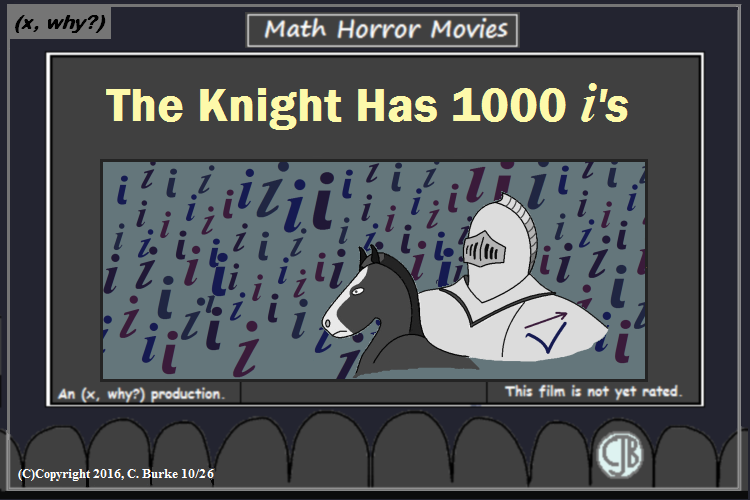One Thousand i's with a Quest to be The One!

Okay, this one isn't a movie, but there is a story. This week, we lost Bobby Vee, a pop idol from before my time, actually. Over the years, I'd heard some of his hits on the Oldies station, whether I knew that they were his at the time is another question.

Anyway, a group of friends were together for Game Night one Saturday evening, and while we're sitting at the table, someone decided to put on the radio for background music. The only song I remember playing was "The Night Has 1000 Eyes", by Bobby Vee. I remember it because it was the first time that any of us had ever heard that oldie, and we thought it was the stupidest thing we'd ever heard -- but in a fun way. We were laughing about it the rest of the evening.

So much so that the next time we all got together at that same house, probably a month or two later, we put the radio back on. And when the song didn't come on, one of the girls picked up the phone -- they were still attached to the wall back then! -- called the station and requested it. She said it was her favorite. And it played a few minutes later. And we sang along as well as we could because we didn't actually know the words.

Life was good.

So R.I.P., Mr. Bobby Vee. Those thousand eyes can't help but see.
Except that C is 100, not 1000.

Video: The Night Has 1000 Eyes

Come back often for more funny math and geeky comics.## Monday, October 24, 2016

### Ninth Anniversary Poem!

(Click on the comic if you can't see the full image.)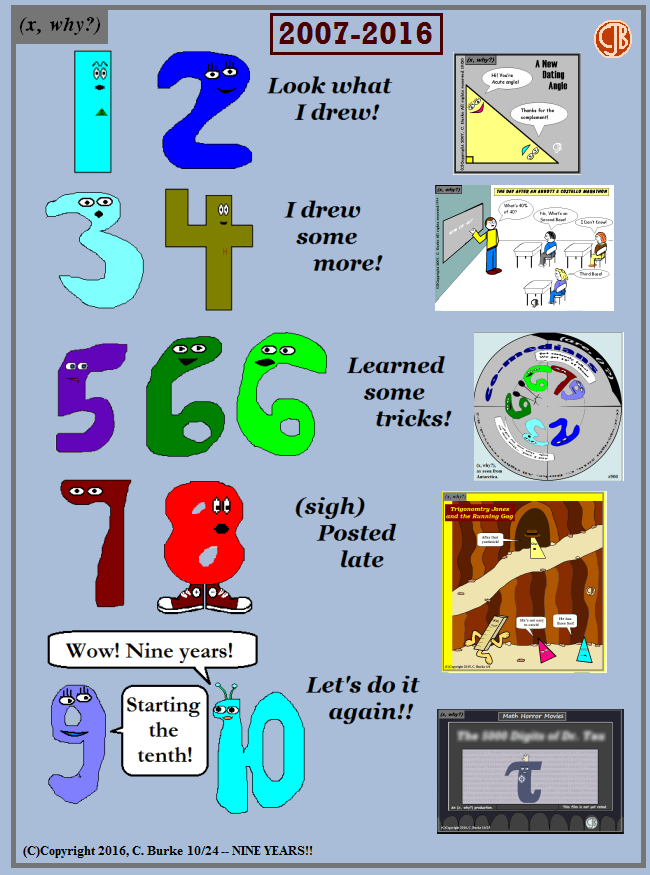Nine Years and my ''side project'' is still going strong.
(for varying values of strong.)

Come back often for more funny math and geeky comics.## Saturday, October 22, 2016

### August 2016 Common Core Algebra II Regents Part 4

I don't usually post the answers to the Algebra II exam, but I've received enough requests that I've decided to post some of the open-ended questions.
Some questions were posted earlier here, here, here and here

### August 2016, Algebra II Part 4

37. Seth’s parents gave him \$5000 to invest for his 16th birthday. He is considering two investment options. Option A will pay him 4.5% interest compounded annually. Option B will pay him 4.6% compounded quarterly.
Write a function of option A and option B that calculates the value of each account after n years.

Seth plans to use the money after he graduates from college in 6 years. Determine how much more money option B will earn than option A to the nearest cent.

Algebraically determine, to the nearest tenth of a year, how long it would take for option B to double Seth’s initial investment.

Not an overly difficult Part 4 question, but it is a little involved.

For the first part, be sure to write a function and not just an expression. The initial amount is \$5000, the rates are 0.045 and 0.046, respectively. Because the second one is quarterly, you have to divide the rate by 4 and multiple the exponent, n, by 4. (You would not be incorrect if you did this in the first function, using 1.)
A = 5000(1 + 0.045)n
B = 5000(1 + 0.046/4)4n

Use the two functions you just wrote, with n = 6. Write down both amounts and then subtract.
A = 5000(1 + 0.045)6 = 6511.30062424 = 6511.30
B = 5000(1 + 0.046/4)(4)(6) = 6578.86985121 = 6578.87
6578.87 - 6511.30 = 67.57

Doubling the \$5000 investment means the future value will be \$10000. Put this in the second function, and solve for n.
10000 = 5000(1 + 0.046/4)4n -- divide both sides by 5000
2 = (1 + 0.046/4)4n -- change the rational expression into a decimal
2 = (1.0115)4n -- solve using logs
4n = log1.01152 -- put this into your calculator
4n = 60.6196... -- divided by 4
n = 15.1549
In 15.2 years, Seth's investment will double.

End of exam

Any questions?

## Friday, October 21, 2016

### Math Horror Movies: The Invisible One

(Click on the comic if you can't see the full image.)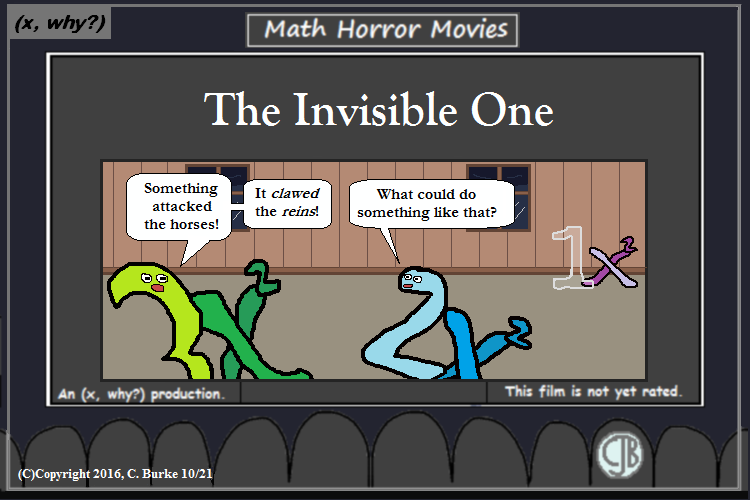He clawed the reins, H.G.! He clawed the reins!

Come back often for more funny math and geeky comics.## Tuesday, October 11, 2016

### (x, why?) Mini: Is the Order Ready?

(Click on the comic if you can't see the full image.)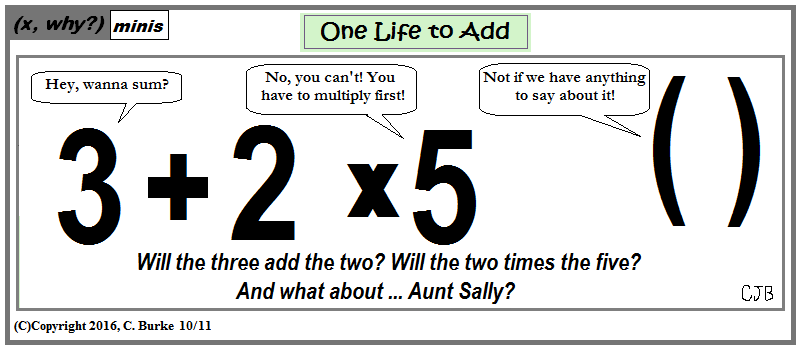Don't be surprised! Parenthesize!

Come back often for more funny math and geeky comics.## Sunday, October 09, 2016

### August 2016 Common Core Algebra II Regents Part 3 (continued)

I don't usually post the answers to the Algebra II exam, but I've received enough requests that I've decided to post some of the open-ended questions.
Some questions were posted earlier here, here and here.

### August 2016, Algebra II Part 3

35. Solve the equation SQRT(2x - 7) + x = 5 algebraically, and justify the solution set.

Look at the image below. The procedure is to subtract x from both sides to isolate the radical expression. Next, square both sides of the equation, leaving 2x - 7 on the left side and a quadratic on the right. Subtract (2x - 7) from both sides to set up the quadratic equation that you are going to solve.

Once you solve it, you must check both answers to see that they are in fact solutions.
You can see from the check that 4 is a solution, but 8 is not, so it is rejected.

36. Ayva designed an experiment to determine the effect of a new energy drink on a group of 20 volunteer students. Ten students were randomly selected to form group 1 while the remaining 10 made up group 2. Each student in group 1 drank one energy drink, and each student in group 2 drank one cola drink. Ten minutes later, their times were recorded for reading the same paragraph of a novel. The results of the experiment are shown below.

a) Ayva thinks drinking energy drinks makes students read faster. Using information from the experimental design or the results, explain why Ayva’s hypothesis may be incorrect.

Using the given results, Ayva randomly mixes the 20 reading times, splits them into two groups of 10, and simulates the difference of the means 232 times.

b) Ayva has decided that the difference in mean reading times is not an unusual occurence. Support her decision using the results of the simulation. Explain your reasoning.

Anya's hypothesis may be incorrect because some of the students in Group 2 read faster than students in Group 1. The means for Groups 1 and 2 are listed as 17.7 and 19.1, respectively. If you find the standard deviation (put the data into Lists in the graphing calculator and do 1-Var Stats on each) you will find the standard deviations for Groups 1 and 2 are 1.396... and 3.720... So there is overlap between the groups.

The difference between the two means is 17.7 - 19.1 = -1.4. Since scores less than this happen 10% of the time, this is not an unusual occurrence.

To be continued

Any questions?

More to come.

## Saturday, October 08, 2016

### August 2016 Common Core Algebra II Regents Part 3

I don't usually post the answers to the Algebra II exam, but I've received enough requests that I've decided to post some of the open-ended questions.
Some questions were posted earlier here, here and here.

### August 2016, Algebra II Part 3

33. Find algebraically the zeros for p(x) = x3 + x2 - 4x - 4.

On the set of axes below, graph y = p(x).

Group the first two terms and the last two terms of the expression. Note that this causes a sign change in the second group:

p(x) = (x3 + x2) - (4x + 4)

Factor each pair:
p(x) = x2(x + 1) - 4(x + 1)

Do you see that each pair has (x + 1) in it? That can be factored, and put up front:
p(x) = (x + 1)(x2 - 4)

Almost done. the x2 expression is the Difference of Squares and can be factored further:
p(x) = (x + 1)(x + 2)(x - 2)

Set p(x) = 0
0 = (x + 1)(x + 2)(x - 2)

(x + 1) = 0 or (x + 2) = 0 or (x - 2) = 0

x = -1 or x = -2 or x = 2

Put the equation in your calculator to get a table of values. From part 1, you know you have these points:
the roots (-1, 0), (-2, 0) and (2, 0) as well as (0, -4), the y-intercept.
You need x = 1. p(1) = (1)3 + (1)2 - 4(1) - 4 = 1 + 1 - 4 - 4 = -6. So (1, -6) is a point on the curve.
Important: You need to see the curve, so use the calculator!

34. One of the medical uses of Iodine–131 (I–131), a radioactive isotope of iodine, is to enhance x-ray images. The half-life of I–131 is approximately 8.02 days. A patient is injected with 20 milligrams of I–131. Determine, to the nearest day, the amount of time needed before the amount of I–131 in the patient’s body is approximately 7 milligrams.

Given the fractions, exponents and subscripts in this problem, I'm going to use an image file to show my work.

Basically, you need to use the formula for exponential decay: y = a(b)x
In this case, y = 7, your target amount, a = 20, the initial amount, b = .5, which is half, and x = t/8.02 days because you are finding the number of 8.02 day increments.
Finding the exponent means using log.5 7/20 = 1.5145731728...
Multiply by 8.02 and round down. The answer is 12 days.

Any questions?

More to come.

## Wednesday, October 05, 2016

### (x, why?) Minis: COPS

(Click on the comic if you can't see the full image.)Have you noticed the shape of the police officers yet?

I've used these cops about 6 times now, starting with a Math Horror Movie comic in 2013 and then using them in the Mini comics. After one of them, I commented that with their "hats" on, those police are actually concave octagons because they have eight sides.

To which I responded, "Keystone Cops?? Who would think of such a thing!"

As an added bonus, I later found out that an old friend of mine read the exchange and immediately slapped his forehead, dropped his jaw and shook his head. He said it was, in retrospect, obvious, but he hadn't seen it coming.

It's nice when there are bonuses for people to find!

By the way, everyone loves "Dad jokes", right?

Come back often for more funny math and geeky comics.## Tuesday, October 04, 2016

### August 2016 Common Core Algebra II Regents Part 2, continued

I don't usually post the answers to the Algebra II exam, but I've received enough requests that I've decided to post some of the open-ended questions.
Some questions were posted earlier here and here.

### August 2016, Algebra II Part 2 (continued)

31. The distance needed to stop a car after applying the brakes varies directly with the square of the car’s speed. The table below shows stopping distances for various speeds.

Determine the average rate of change in braking distance, in ft/mph, between one car traveling at 50 mph and one traveling at 70 mph.
Explain what this rate of change means as it relates to braking distance.

32. Given events A and B, such that P(A) = 0.6, P(B) = 0.5, and P(A ∪ B) = 0.8, determine whether A and B are independent or dependent.

P(A ∪ B) = P(A) + P(B) - P(A ∩ B).
0.8 = 0.6 + 0.5 - P(A ∩ B)
0.8 = 1.1 - P(A ∩ B)
-0.3 = - P(A ∩ B)
0.3 = P(A ∩ B)

If A and B are independent, then P(A ∩ B) = P(A) * P(B)
0.3 = (0.6)(0.5)
0.3 = 0.3
Therefore, A and B are independent.

End of Part II.

to be continued.

Any questions?